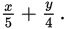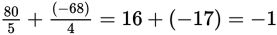# SAT Math Multiple Choice Question 418: Answer and Explanation

### Test Information

Question: 418

13. If y = 12 - x and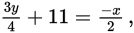what is the value of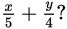• A. -1
• B.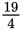• C.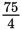• D. 33

Explanation:

A

Difficulty: Hard

Category: Heart of Algebra / Systems of Linear Equations

Strategic Advice: Don't let all the fractions intimidate you. There are two equations and two variables, so solve this system the same way you would solve any other system of equations.

Getting to the Answer: The first equation is already solved perfectly for y, so use substitution. To make the second equation easier to work with, multiply it by 4 to clear the fractions (even though you may have noticed the tempting 4 in the denominator of the desired expression).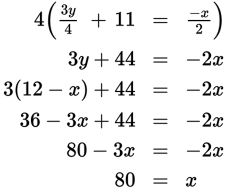Next, substitute 80 for x into the first equation and solve for y.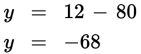Finally, substitute the values you found into the expression in the question,